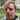# Why is 0.1 + 0.2 weird in JavaScript?

December 30, 2020

# What’s the problem?

Just open the dev console in your browser right now and type in 0.1 + 0.2. Suprised to discover the result is 0.30000000000000004? What’s that about?

# How does math work in JavaScript?

Actually this behaviour comes from computer architecture in general - it’s not specific to JavaScript. I’ll give you a kindergarten breakdown but consider there’s lots of number theory/computer science involved here.

In a nutshell computers are designed around binary numbers whereas humans do math in a decimal system.

When you have 0.1 + 0.2 in your code - the computer has to take those numbers, translate them to binary, do the math, transform the result to decimal again and then giving it back to you.

Some fractions don’t have precise values in each system - they only have approximations. Consider 1/3 in decimal - the result 0.3333(3) is an approximation - you can keep adding 3s to its tail, increasing the precision. But it will never be an exact value because a decmial system is based on the number 10 and 10 things can never be divided up in 3 equal piles (you might be visualzing a bunch of apples at this point?).

The same thing happens in binary. The Binary system is, of course, based on the number 2 and thus we’re always working with a groups of 2 things. Binary is all about halves - the fraction 1/2 in binary has an exact value becuase 1/2 means “half of the things” and half of 2 things is 1 thing. Easy peasy.

3/10 on the other hand is a problem. It works great in decimal where’s we’re always thinking in 10s - take 3 of the 10 things. Because 3/10 is not half of a thing - it lacks a percise representation in binary. Instead, its value is an approximation of the value 0.3 in decimal. When you ask the computer to calculate 0.1 + 0.2 for you - the result 0.3 is converted from binary (where it doesn’t have a precise value) to decimal. The equivalent value is 0.30000000000000004. This is a close approximation of 0.3 in decimal but it’s not quite the same value.

As you’ve probably already inferred: that this is not specific to 0.1 + 0.2. Other operation resulting in a number lacking a precise representation in binary will hit the same thing.

# Should you be concerned with this?

Depends on what you’re doing, I guess. In my work I’m (mostly) pumping JSON in and out of forms - not much math involved. I rarely (if ever) use foating point numbers and all of this is mostly a curiousity. Hence, I’m not suggestion solutions.

This imprecision is a big deal if, on the other hand, you’re doing rocket science 🚀 - calculcating launch trajectories and whatnot. But if so - what are you doing reading this blog? - it’s explained better elsewhere.I'm Stoil Stoychev, a frontend software engineer, working with React and GraphQL. This blog (mostly) explores my tech stack.